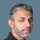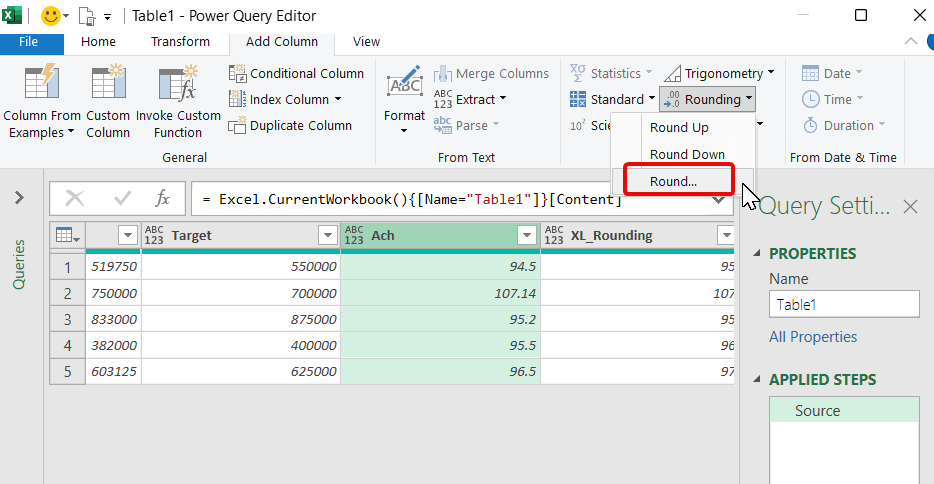cancel
Showing results for
Did you mean:## Excel vs Power Query: The Rounding Dilemma

Have you ever encountered an issue where your calculations in Excel and Power Query don’t match up due to the way rounding is handled? Rounding is a crucial aspect of financial calculations, and inconsistent results between Excel and Power Query can lead to costly mistakes.

Let’s take a look at an example. Say you have a table of employee sales data, including their actual sales, target sales, and achievement percentages. If an employee achieves their target sales by rounding 95% or above, they’re eligible for a sales commission.In this example, employee A has achieved 94.5% of their target sales. When rounded using the Excel Round function, the result is correctly rounded to 95% and A becomes eligible. However, the same calculation in Power Query results in a rounded value of 94%. and he isn’t eligible for commission.

So, what’s going on here? The difference in results is due to the way Excel and Power Query handle rounding.

Excel uses the “Round half away from zero” method of rounding, which means that any value of 0.5 or greater is rounded up to the nearest whole number, and any value less than 0.5 is rounded down to the nearest whole number. In contrast, Power Query uses the “Round half to even” method of rounding, also known as banker’s rounding. This method rounds values to the nearest even number if the value in the decimal place is exactly 0.5. For example, 1.5 is rounded to 2, but 2.5 is rounded to 2.

In our example, the nearest even number to 94.5 is 94, so Power Query rounds the value down to 94. On the other hand, Excel correctly rounds the value up to 95.

To ensure consistent rounding results between Excel and Power Query, we can make a small adjustment to the Round function in Power Query. The Number.Round function in Power Query has a third argument value called “RoundingMode.AwayFromZero” This argument can be added to the function to force Power Query to use the “Round half away from zero” method of rounding, just like Excel.I imported the data from Excel to Power Query, add a new column based on “Ach” column  with the application of simple roundingSet Decimal Places to zeroModifed the Number.Round function in Power Query to include the third argument “RoundingMode.AwayFromZero” to achieve consistent results with Excel.

As you can see, the Round function in Power Query now produces the same results as Excel, ensuring consistency in our calculations.

By adding the third argument, we are instructing Power Query to round the value to the nearest whole number away from zero, which ensures that values of 0.5 or greater are rounded up to the nearest whole number, just like in Excel.

In conclusion, rounding is an essential aspect of financial calculations, and inconsistent rounding results between Excel and Power Query can lead to costly mistakes. By understanding the difference in how Excel and Power Query handle rounding, we can make the necessary adjustments to ensure consistent results. By modifying the Round function in Power Query to use the “Round half away from zero” method of rounding, we can achieve consistency in our calculations with Excel.

So next time you’re working with financial data in Power Query, remember to pay attention to the rounding method and make the necessary adjustments to ensure consistent and accurate results.

Top Kudoed Posts
Latest Articles